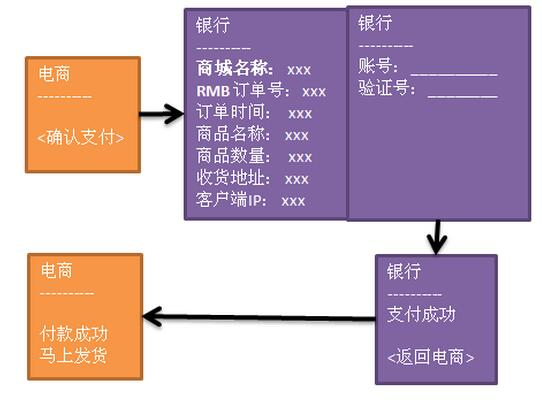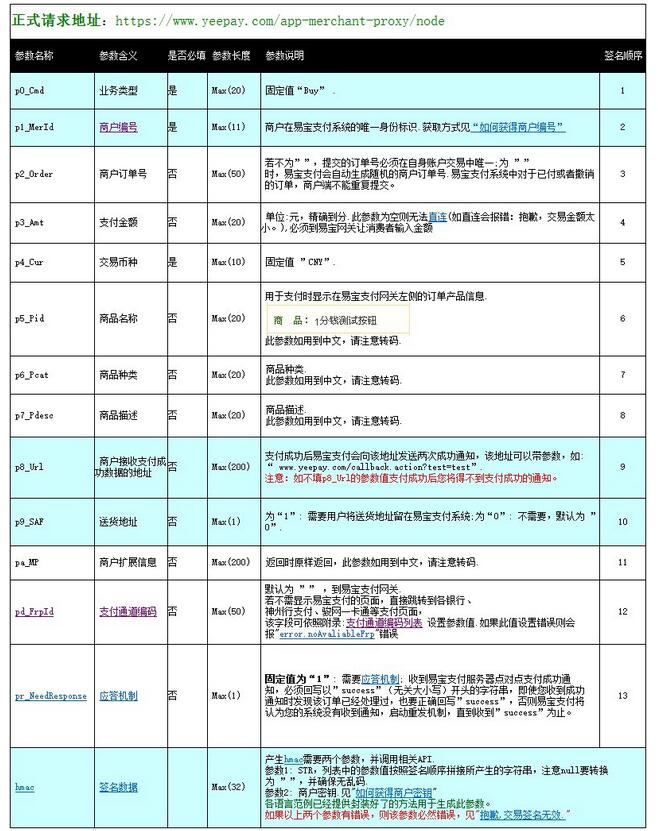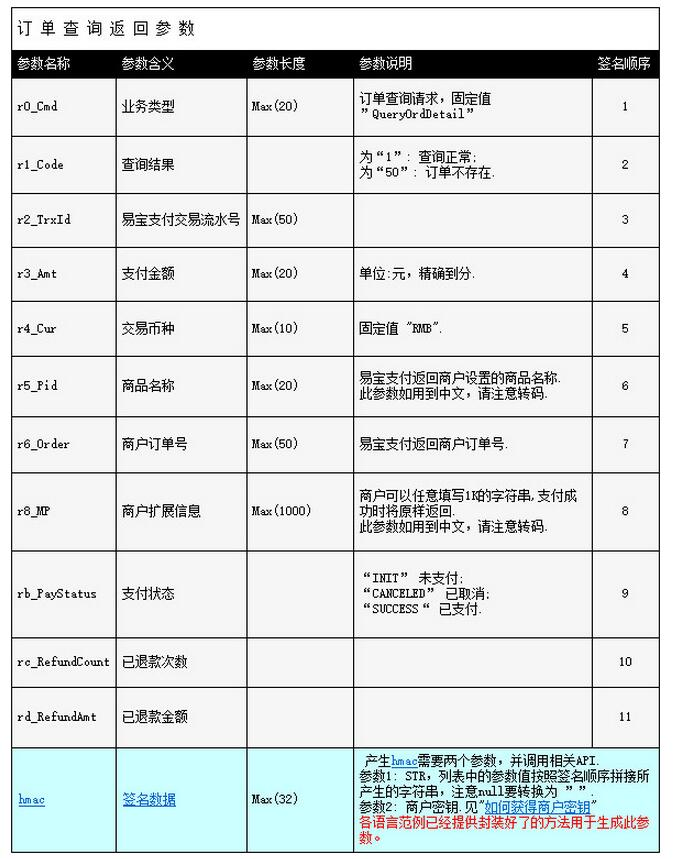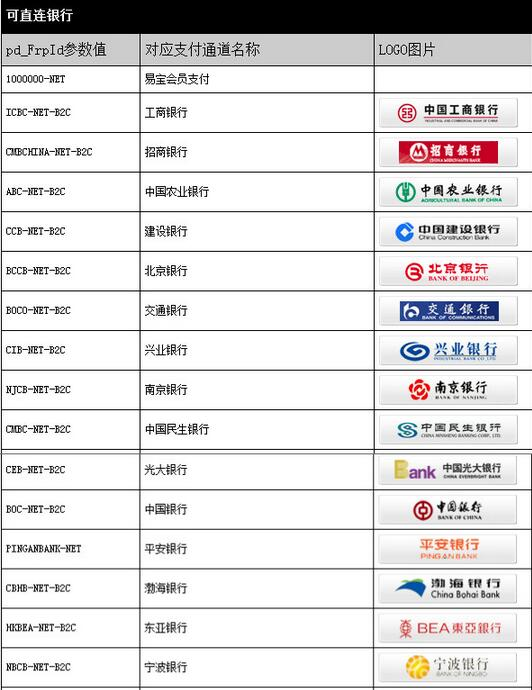# javaweb实现在线支付功能

2020-02-14 14:03

1、在线支付概述

2、两种在线支付的方式

*电商直接与银行对接
*电商通过第三方支付平台与银行对接3、通过第三方在线支付规则4、开发第三方在线支付系统

BackServlet：当用户支付成功后，第三方会重定向到我们指定的返回页面，我们使用BackServlet作为返回页面，它用来接收第三方传递的参数，显示在页面中

`<form action="" method="post"> 订单号：<input type="text" name="p2_Order"/><br/> 金　额：<input type="text" name="p3_Amt"/><br/> 选择银行：<br/> <input type="radio" name="pd_FrpId" value="ICBC-NET-B2C"/>工商银行 <img src="bank_img/icbc.bmp" align="middle"/> <input type="radio" name="pd_FrpId" value="BOC-NET-B2C"/>中国银行 <img src="bank_img/bc.bmp" align="middle"/><br/><br/> <input type="radio" name="pd_FrpId" value="ABC-NET-B2C"/>农业银行 <img src="bank_img/abc.bmp" align="middle"/> <input type="radio" name="pd_FrpId" value="CCB-NET-B2C"/>建设银行 <img src="bank_img/ccb.bmp" align="middle"/><br/><br/> <input type="radio" name="pd_FrpId" value="BOCO-NET-B2C"/>交通银行 <img src="bank_img/bcc.bmp" align="middle"/><br/> <input type="submit" value="确认支付"/></form>``public class BuyServlet extends HttpServlet { public void doPost(HttpServletRequest request, HttpServletResponse response)   throws ServletException, IOException {  request.setCharacterEncoding("utf-8");  response.setContentType("text/html;charset=utf-8");  String p0_Cmd = "Buy";// 业务类型，固定值为buy，即“买”  String p1_MerId = "10001126856";// 在易宝注册的商号  String p2_Order = request.getParameter("p2_Order");// 订单编号  String p3_Amt = request.getParameter("p3_Amt");// 支付的金额  String p4_Cur = "CNY";// 交易种币，固定值为CNY，表示人民币  String p5_Pid = "";// 商品名称  String p6_Pcat = "";// 商品各类  String p7_Pdesc = "";// 商品描述  String p8_Url = "http://localhost:8080/buy/BackServlet";// 电商的返回页面，当支付成功后，易宝会重定向到这个页面  String p9_SAF = "";// 送货地址  String pa_MP = "";// 商品扩展信息  String pd_FrpId = request.getParameter("pd_FrpId");// 支付通道，即选择银行  String pr_NeedResponse = "1";// 应答机制，固定值为1  // 密钥，由易宝提供，只有商户和易宝知道这个密钥。  String keyValue = "69cl522AV6q613Ii4W6u8K6XuW8vM1N6bFgyv769220IuYe9u37N4y7rI4Pl";  // 通过上面的参数、密钥、加密算法，生成hmac值  // 参数的顺序是必须的，如果没有值也不能给出null，而应该给出空字符串。  String hmac = PaymentUtil.buildHmac(p0_Cmd, p1_MerId, p2_Order, p3_Amt,    p4_Cur, p5_Pid, p6_Pcat, p7_Pdesc, p8_Url, p9_SAF, pa_MP,    pd_FrpId, pr_NeedResponse, keyValue);  // 把所有参数连接到网关地址后面  String url = "https://www.yeepay.com/app-merchant-proxy/node";  url += "?p0_Cmd=" + p0_Cmd +     "&p1_MerId=" + p1_MerId +    "&p2_Order=" + p2_Order +     "&p3_Amt=" + p3_Amt +     "&p4_Cur=" + p4_Cur +     "&p5_Pid=" + p5_Pid +     "&p6_Pcat=" + p6_Pcat +     "&p7_Pdesc=" + p7_Pdesc +     "&p8_Url=" + p8_Url +     "&p9_SAF=" + p9_SAF +     "&pa_MP=" + pa_MP +     "&pd_FrpId=" + pd_FrpId +     "&pr_NeedResponse=" + pr_NeedResponse +     "&hmac=" + hmac;  System.out.println(url);  // 重定向到网关  response.sendRedirect(url); }}`

`public class BackServlet extends HttpServlet { public void doGet(HttpServletRequest request, HttpServletResponse response)   throws ServletException, IOException {  response.setContentType("text/html;charset=utf-8");  /*   * 易宝会提供一系列的结果参数，我们获取其中需要的即可   * 获取支付结果：r1_Code，1表示支付成功。   * 获取支付金额：r3_Amt   * 获取电商的订单号：r6_Order   * 获取结果返回类型：r9_BType，1表示重定向返回，2表示点对点返回，   *  但点对点我们收不到，因为我们的ip都是局域网ip。   */  String r1_Code = request.getParameter("r1_Code");  String r3_Amt = request.getParameter("r3_Amt");  String r6_Order = request.getParameter("r6_Order");  String r9_BType = request.getParameter("r9_BType");  if(r1_Code.equals("1")) {   if(r9_BType.equals("1")) {    response.getWriter().print("<h1>支付成功！</h1>");//其实支付不成功时根本易宝根本就不会返回到本Servlet    response.getWriter().print("支付金额为：" + r3_Amt + "<br/>");    response.getWriter().print("订单号为：" + r6_Order + "<br/>");   }  } }}`

`public class PaymentUtil { private static String encodingCharset = "UTF-8"; /**  * 生成hmac方法  *   * @param p0_Cmd 业务类型  * @param p1_MerId 商户编号  * @param p2_Order 商户订单号  * @param p3_Amt 支付金额  * @param p4_Cur 交易币种  * @param p5_Pid 商品名称  * @param p6_Pcat 商品种类  * @param p7_Pdesc 商品描述  * @param p8_Url 商户接收支付成功数据的地址  * @param p9_SAF 送货地址  * @param pa_MP 商户扩展信息  * @param pd_FrpId 银行编码  * @param pr_NeedResponse 应答机制  * @param keyValue 商户密钥  * @return  */ public static String buildHmac(String p0_Cmd,String p1_MerId,   String p2_Order, String p3_Amt, String p4_Cur,String p5_Pid, String p6_Pcat,   String p7_Pdesc,String p8_Url, String p9_SAF,String pa_MP,String pd_FrpId,   String pr_NeedResponse,String keyValue) {  StringBuilder sValue = new StringBuilder();  // 业务类型  sValue.append(p0_Cmd);  // 商户编号  sValue.append(p1_MerId);  // 商户订单号  sValue.append(p2_Order);  // 支付金额  sValue.append(p3_Amt);  // 交易币种  sValue.append(p4_Cur);  // 商品名称  sValue.append(p5_Pid);  // 商品种类  sValue.append(p6_Pcat);  // 商品描述  sValue.append(p7_Pdesc);  // 商户接收支付成功数据的地址  sValue.append(p8_Url);  // 送货地址  sValue.append(p9_SAF);  // 商户扩展信息  sValue.append(pa_MP);  // 银行编码  sValue.append(pd_FrpId);  // 应答机制  sValue.append(pr_NeedResponse);  return PaymentUtil.hmacSign(sValue.toString(), keyValue); } /**  * 返回校验hmac方法  *   * @param hmac 支付网关发来的加密验证码  * @param p1_MerId 商户编号  * @param r0_Cmd 业务类型  * @param r1_Code 支付结果  * @param r2_TrxId 易宝支付交易流水号  * @param r3_Amt 支付金额  * @param r4_Cur 交易币种  * @param r5_Pid 商品名称  * @param r6_Order 商户订单号  * @param r7_Uid 易宝支付会员ID  * @param r8_MP 商户扩展信息  * @param r9_BType 交易结果返回类型  * @param keyValue 密钥  * @return  */ public static boolean verifyCallback(String hmac, String p1_MerId,   String r0_Cmd, String r1_Code, String r2_TrxId, String r3_Amt,   String r4_Cur, String r5_Pid, String r6_Order, String r7_Uid,   String r8_MP, String r9_BType, String keyValue) {  StringBuilder sValue = new StringBuilder();  // 商户编号  sValue.append(p1_MerId);  // 业务类型  sValue.append(r0_Cmd);  // 支付结果  sValue.append(r1_Code);  // 易宝支付交易流水号  sValue.append(r2_TrxId);  // 支付金额  sValue.append(r3_Amt);  // 交易币种  sValue.append(r4_Cur);  // 商品名称  sValue.append(r5_Pid);  // 商户订单号  sValue.append(r6_Order);  // 易宝支付会员ID  sValue.append(r7_Uid);  // 商户扩展信息  sValue.append(r8_MP);  // 交易结果返回类型  sValue.append(r9_BType);  String sNewString = PaymentUtil.hmacSign(sValue.toString(), keyValue);  return sNewString.equals(hmac); } /**  * @param aValue  * @param aKey  * @return  */ public static String hmacSign(String aValue, String aKey) {  byte k_ipad[] = new byte;  byte k_opad[] = new byte;  byte keyb[];  byte value[];  try {   keyb = aKey.getBytes(encodingCharset);   value = aValue.getBytes(encodingCharset);  } catch (UnsupportedEncodingException e) {   keyb = aKey.getBytes();   value = aValue.getBytes();  }  Arrays.fill(k_ipad, keyb.length, 64, (byte) 54);  Arrays.fill(k_opad, keyb.length, 64, (byte) 92);  for (int i = 0; i < keyb.length; i++) {   k_ipad[i] = (byte) (keyb[i] ^ 0x36);   k_opad[i] = (byte) (keyb[i] ^ 0x5c);  }  MessageDigest md = null;  try {   md = MessageDigest.getInstance("MD5");  } catch (NoSuchAlgorithmException e) {   return null;  }  md.update(k_ipad);  md.update(value);  byte dg[] = md.digest();  md.reset();  md.update(k_opad);  md.update(dg, 0, 16);  dg = md.digest();  return toHex(dg); } public static String toHex(byte input[]) {  if (input == null)   return null;  StringBuffer output = new StringBuffer(input.length * 2);  for (int i = 0; i < input.length; i++) {   int current = input[i] & 0xff;   if (current < 16)    output.append("0");   output.append(Integer.toString(current, 16));  }  return output.toString(); } /**  *   * @param args  * @param key  * @return  */ public static String getHmac(String[] args, String key) {  if (args == null || args.length == 0) {   return (null);  }  StringBuffer str = new StringBuffer();  for (int i = 0; i < args.length; i++) {   str.append(args[i]);  }  return (hmacSign(str.toString(), key)); } /**  * @param aValue  * @return  */ public static String digest(String aValue) {  aValue = aValue.trim();  byte value[];  try {   value = aValue.getBytes(encodingCharset);  } catch (UnsupportedEncodingException e) {   value = aValue.getBytes();  }  MessageDigest md = null;  try {   md = MessageDigest.getInstance("SHA");  } catch (NoSuchAlgorithmException e) {   e.printStackTrace();   return null;  }  return toHex(md.digest(value)); }// public static void main(String[] args) {//  System.out.println(hmacSign("AnnulCard1000043252120080620160450.0http://localhost/SZXpro/callback.asp杩?4564868265473632445648682654736324511","8UPp0KE8sq73zVP370vko7C39403rtK1YwX40Td6irH216036H27Eb12792t"));// }}`

hmac：13参数值+keyValue(密钥) + 算法(md5)

13参数值：自己设置的！
keyValue：易宝在我们注册后发给我们的，这个东东只有我们和易宝知道！